Publicité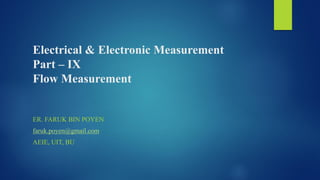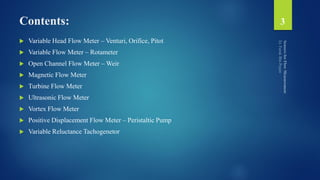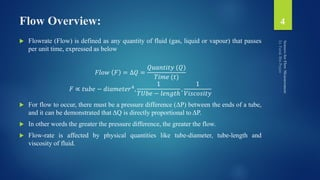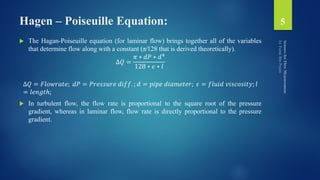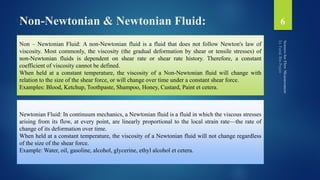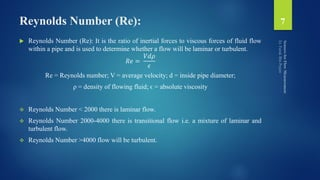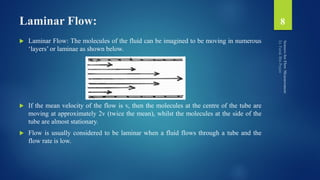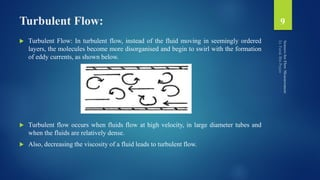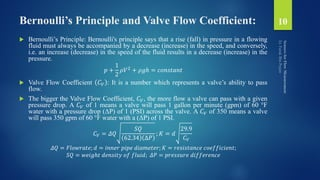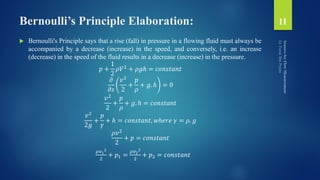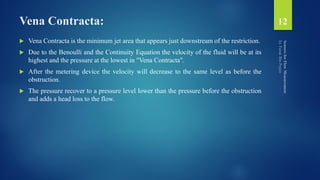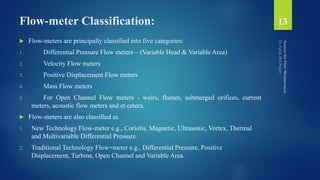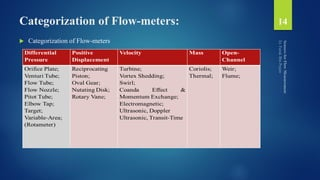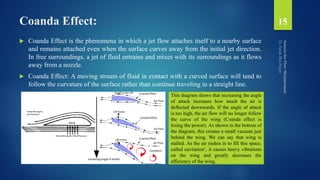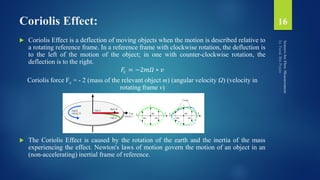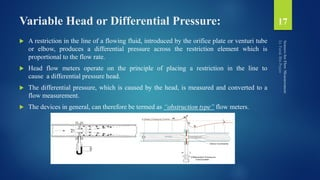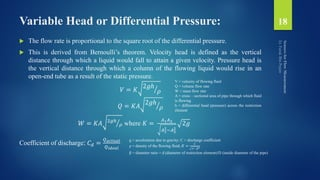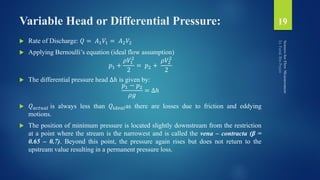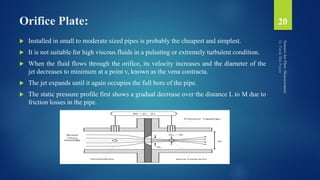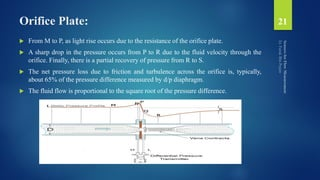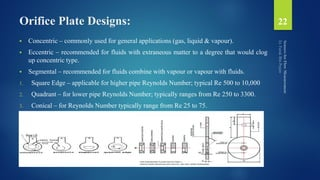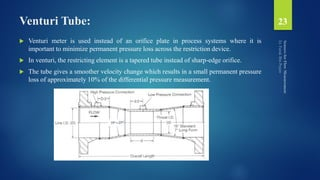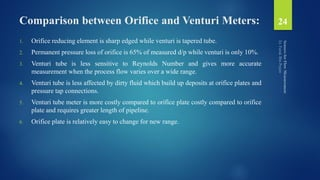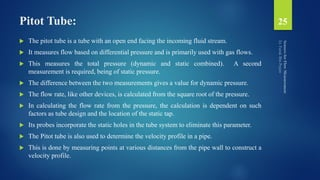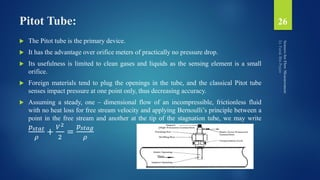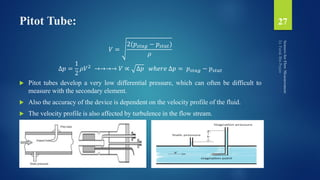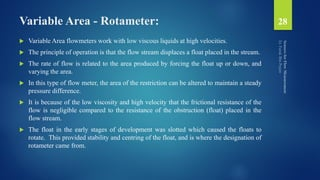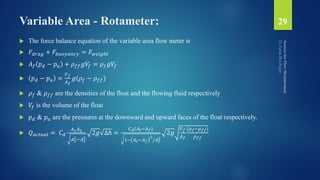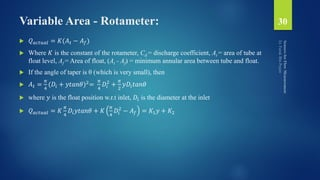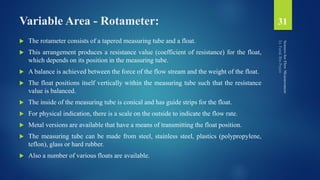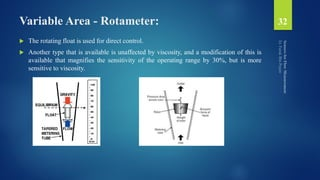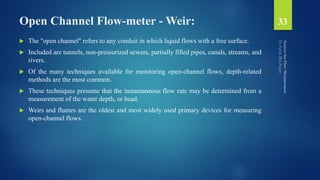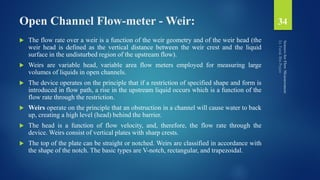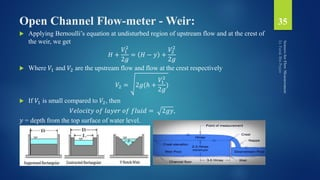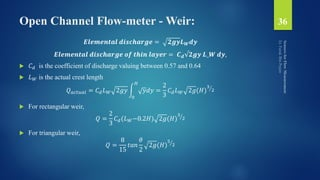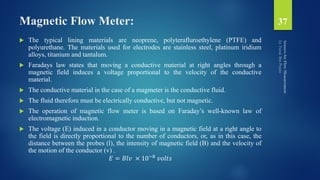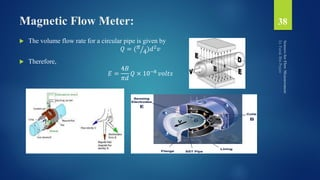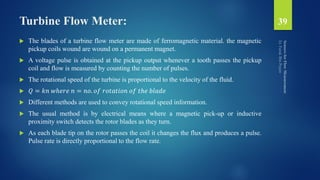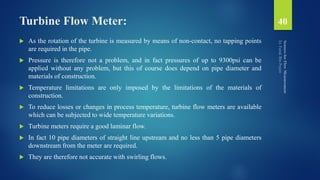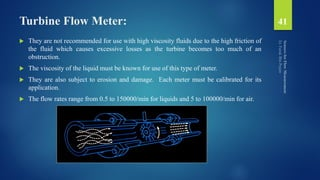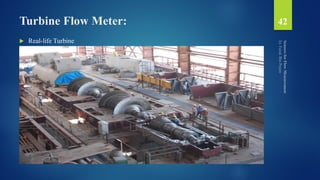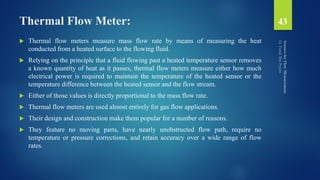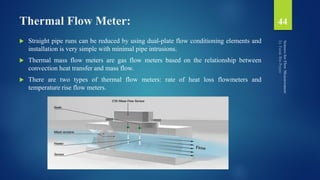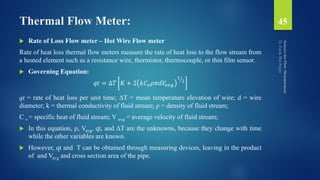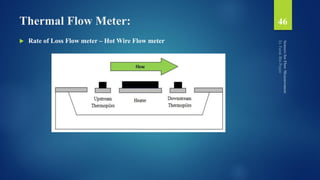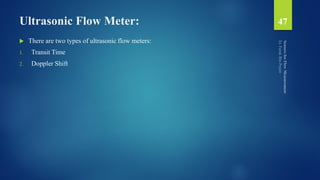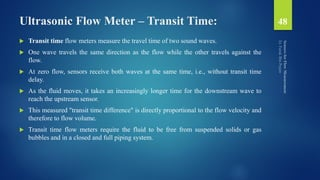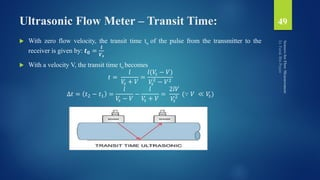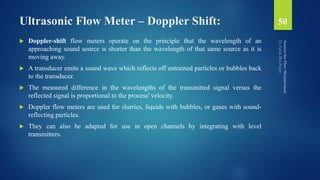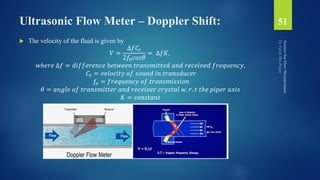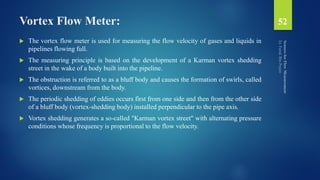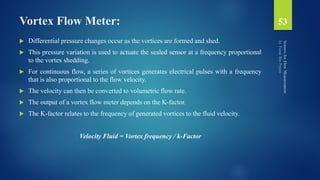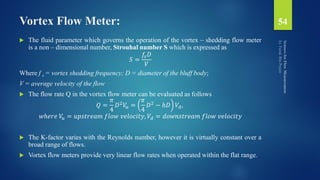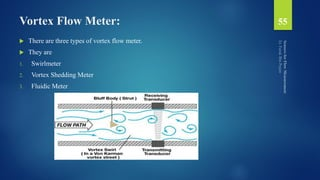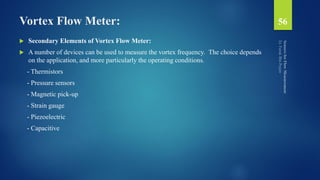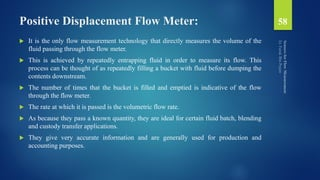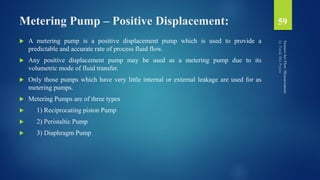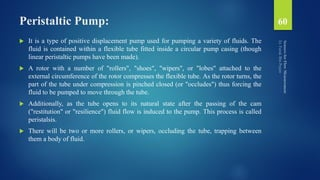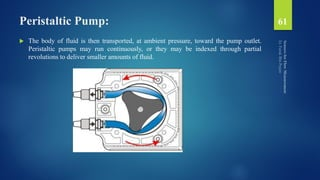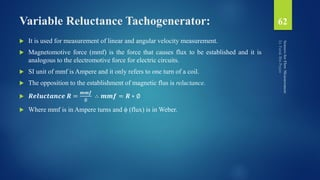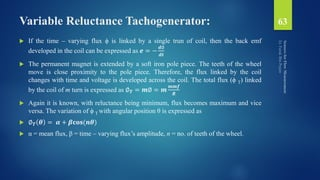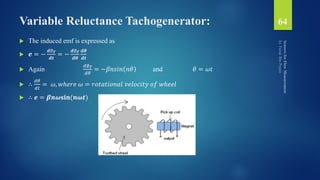1 sur 64
Publicité

### Electronic Measurement Flow Measurement

1. Electrical & Electronic Measurement Part – IX Flow Measurement ER. FARUK BIN POYEN faruk.poyen@gmail.com AEIE, UIT, BU
2. Contents:  Flow Overview  Hagan-Poiseuille Equation  Fluid Types  Reynolds Number  Flow Types  Bernoulli’s Principle  Flow-meter Classification  Coanda Effect  Coriolis Effect 2
3. Contents:  Variable Head Flow Meter – Venturi, Orifice, Pitot  Variable Flow Meter – Rotameter  Open Channel Flow Meter – Weir  Magnetic Flow Meter  Turbine Flow Meter  Ultrasonic Flow Meter  Vortex Flow Meter  Positive Displacement Flow Meter – Peristaltic Pump  Variable Reluctance Tachogenetor 3
4. Flow Overview:  Flowrate (Flow) is defined as any quantity of fluid (gas, liquid or vapour) that passes per unit time, expressed as below 𝐹𝑙𝑜𝑤 𝐹 = ∆𝑄 = 𝑄𝑢𝑎𝑛𝑡𝑖𝑡𝑦 (𝑄) 𝑇𝑖𝑚𝑒 (𝑡) 𝐹 ∝ 𝑡𝑢𝑏𝑒 − 𝑑𝑖𝑎𝑚𝑒𝑡𝑒𝑟4, 1 𝑇𝑈𝑏𝑒 − 𝑙𝑒𝑛𝑔𝑡ℎ , 1 𝑉𝑖𝑠𝑐𝑜𝑠𝑖𝑡𝑦  For flow to occur, there must be a pressure difference (ΔP) between the ends of a tube, and it can be demonstrated that ΔQ is directly proportional to ΔP.  In other words the greater the pressure difference, the greater the flow.  Flow-rate is affected by physical quantities like tube-diameter, tube-length and viscosity of fluid. 4
5. Hagen – Poiseuille Equation:  The Hagan-Poiseuille equation (for laminar flow) brings together all of the variables that determine flow along with a constant (π⁄128 that is derived theoretically). ∆𝑄 = 𝜋 ∗ 𝑑𝑃 ∗ 𝑑4 128 ∗ 𝜖 ∗ 𝑙 ∆𝑄 = 𝐹𝑙𝑜𝑤𝑟𝑎𝑡𝑒; 𝑑𝑃 = 𝑃𝑟𝑒𝑠𝑠𝑢𝑟𝑒 𝑑𝑖𝑓𝑓. ; 𝑑 = 𝑝𝑖𝑝𝑒 𝑑𝑖𝑎𝑚𝑒𝑡𝑒𝑟; 𝜖 = 𝑓𝑙𝑢𝑖𝑑 𝑣𝑖𝑠𝑐𝑜𝑠𝑖𝑡𝑦; 𝑙 = 𝑙𝑒𝑛𝑔𝑡ℎ;  In turbulent flow, the flow rate is proportional to the square root of the pressure gradient, whereas in laminar flow, flow rate is directly proportional to the pressure gradient. 5
6. Non-Newtonian & Newtonian Fluid: 6 Non – Newtonian Fluid: A non-Newtonian fluid is a fluid that does not follow Newton's law of viscosity. Most commonly, the viscosity (the gradual deformation by shear or tensile stresses) of non-Newtonian fluids is dependent on shear rate or shear rate history. Therefore, a constant coefficient of viscosity cannot be defined. When held at a constant temperature, the viscosity of a Non-Newtonian fluid will change with relation to the size of the shear force, or will change over time under a constant shear force. Examples: Blood, Ketchup, Toothpaste, Shampoo, Honey, Custard, Paint et cetera. Newtonian Fluid: In continuum mechanics, a Newtonian fluid is a fluid in which the viscous stresses arising from its flow, at every point, are linearly proportional to the local strain rate—the rate of change of its deformation over time. When held at a constant temperature, the viscosity of a Newtonian fluid will not change regardless of the size of the shear force. Example: Water, oil, gasoline, alcohol, glycerine, ethyl alcohol et cetera.
7. Reynolds Number (Re):  Reynolds Number (Re): It is the ratio of inertial forces to viscous forces of fluid flow within a pipe and is used to determine whether a flow will be laminar or turbulent. 𝑅𝑒 = 𝑉𝑑𝜌 𝜖 Re = Reynolds number; V = average velocity; d = inside pipe diameter; ρ = density of flowing fluid; ϵ = absolute viscosity  Reynolds Number < 2000 there is laminar flow.  Reynolds Number 2000-4000 there is transitional flow i.e. a mixture of laminar and turbulent flow.  Reynolds Number >4000 flow will be turbulent. 7
8. Laminar Flow:  Laminar Flow: The molecules of the fluid can be imagined to be moving in numerous ‘layers’ or laminae as shown below.  If the mean velocity of the flow is v, then the molecules at the centre of the tube are moving at approximately 2v (twice the mean), whilst the molecules at the side of the tube are almost stationary.  Flow is usually considered to be laminar when a fluid flows through a tube and the flow rate is low. 8
9. Turbulent Flow:  Turbulent Flow: In turbulent flow, instead of the fluid moving in seemingly ordered layers, the molecules become more disorganised and begin to swirl with the formation of eddy currents, as shown below.  Turbulent flow occurs when fluids flow at high velocity, in large diameter tubes and when the fluids are relatively dense.  Also, decreasing the viscosity of a fluid leads to turbulent flow. 9
10. Bernoulli’s Principle and Valve Flow Coefficient:  Bernoulli’s Principle: Bernoulli's principle says that a rise (fall) in pressure in a flowing fluid must always be accompanied by a decrease (increase) in the speed, and conversely, i.e. an increase (decrease) in the speed of the fluid results in a decrease (increase) in the pressure. 𝑝 + 1 2 𝜌𝑉2 + 𝜌𝑔ℎ = 𝑐𝑜𝑛𝑠𝑡𝑎𝑛𝑡  Valve Flow Coefficient 𝐶 𝑉 : It is a number which represents a valve’s ability to pass flow.  The bigger the Valve Flow Coefficient, 𝐶 𝑉, the more flow a valve can pass with a given pressure drop. A 𝐶 𝑉 of 1 means a valve will pass 1 gallon per minute (gpm) of 60 °F water with a pressure drop (ΔP) of 1 (PSI) across the valve. A 𝐶 𝑉 of 350 means a valve will pass 350 gpm of 60 °F water with a (ΔP) of 1 PSI. 𝐶 𝑉 = 𝛥𝑄 𝑆𝑄 62.34 ∆𝑃 ; 𝐾 = 𝑑 29.9 𝐶 𝑉 𝛥𝑄 = 𝐹𝑙𝑜𝑤𝑟𝑎𝑡𝑒; 𝑑 = 𝑖𝑛𝑛𝑒𝑟 𝑝𝑖𝑝𝑒 𝑑𝑖𝑎𝑚𝑒𝑡𝑒𝑟; 𝐾 = 𝑟𝑒𝑠𝑖𝑠𝑡𝑎𝑛𝑐𝑒 𝑐𝑜𝑒𝑓𝑓𝑖𝑐𝑖𝑒𝑛𝑡; 𝑆𝑄 = 𝑤𝑒𝑖𝑔ℎ𝑡 𝑑𝑒𝑛𝑠𝑖𝑡𝑦 𝑜𝑓 𝑓𝑙𝑢𝑖𝑑; 𝛥𝑃 = 𝑝𝑟𝑒𝑠𝑠𝑢𝑟𝑒 𝑑𝑖𝑓𝑓𝑒𝑟𝑒𝑛𝑐𝑒 10
11. Bernoulli’s Principle Elaboration:  Bernoulli's Principle says that a rise (fall) in pressure in a flowing fluid must always be accompanied by a decrease (increase) in the speed, and conversely, i.e. an increase (decrease) in the speed of the fluid results in a decrease (increase) in the pressure. 𝑝 + 1 2 𝜌𝑉2 + 𝜌𝑔ℎ = 𝑐𝑜𝑛𝑠𝑡𝑎𝑛𝑡 𝜕 𝜕𝑠 𝑣2 2 + 𝑝 𝜌 + 𝑔. ℎ = 0 𝑣2 2 + 𝑝 𝜌 + 𝑔. ℎ = 𝑐𝑜𝑛𝑠𝑡𝑎𝑛𝑡 𝑣2 2𝑔 + 𝑝 𝛾 + ℎ = 𝑐𝑜𝑛𝑠𝑡𝑎𝑛𝑡, 𝑤ℎ𝑒𝑟𝑒 𝛾 = 𝜌. 𝑔 𝜌𝑣2 2 + 𝑝 = 𝑐𝑜𝑛𝑠𝑡𝑎𝑛𝑡 𝜌𝑣1 2 2 + 𝑝1 = 𝜌𝑣2 2 2 + 𝑝2 = 𝑐𝑜𝑛𝑠𝑡𝑎𝑛𝑡 11
12. Vena Contracta:  Vena Contracta is the minimum jet area that appears just downstream of the restriction.  Due to the Benoulli and the Continuity Equation the velocity of the fluid will be at its highest and the pressure at the lowest in "Vena Contracta".  After the metering device the velocity will decrease to the same level as before the obstruction.  The pressure recover to a pressure level lower than the pressure before the obstruction and adds a head loss to the flow. 12
13. Flow-meter Classification:  Flow-meters are principally classified into five categories: 1. Differential Pressure Flow meters – (Variable Head & Variable Area) 2. Velocity Flow meters 3. Positive Displacement Flow meters 4. Mass Flow meters 5. For Open Channel Flow meters - weirs, flumes, submerged orifices, current meters, acoustic flow meters and et cetera.  Flow-meters are also classified as 1. New Technology Flow-meter e.g., Coriolis, Magnetic, Ultrasonic, Vortex, Thermal and Multivariable Differential Pressure. 2. Traditional Technology Flow=meter e.g., Differential Pressure, Positive Displacement, Turbine, Open Channel and Variable Area. 13
14. Categorization of Flow-meters:  Categorization of Flow-meters 14
15. Coanda Effect:  Coanda Effect is the phenomena in which a jet flow attaches itself to a nearby surface and remains attached even when the surface curves away from the initial jet direction. In free surroundings, a jet of fluid entrains and mixes with its surroundings as it flows away from a nozzle.  Coanda Effect: A moving stream of fluid in contact with a curved surface will tend to follow the curvature of the surface rather than continue traveling in a straight line. 15 This diagram shows that increasing the angle of attack increases how much the air is deflected downwards. If the angle of attack is too high, the air flow will no longer follow the curve of the wing (Coanda effect is losing the power). As shown in the bottom of the diagram, this creates a small vacuum just behind the wing. We can say that wing is stalled. As the air rushes in to fill this space, called cavitation’, it causes heavy vibrations on the wing and greatly decreases the efficiency of the wing.
16. Coriolis Effect:  Coriolis Effect is a deflection of moving objects when the motion is described relative to a rotating reference frame. In a reference frame with clockwise rotation, the deflection is to the left of the motion of the object; in one with counter-clockwise rotation, the deflection is to the right. 𝐹𝑐 = −2𝑚𝛺 ∗ 𝑣 Coriolis force Fc = - 2 (mass of the relevant object m) (angular velocity Ω) (velocity in rotating frame v)  The Coriolis Effect is caused by the rotation of the earth and the inertia of the mass experiencing the effect. Newton's laws of motion govern the motion of an object in an (non-accelerating) inertial frame of reference. 16
17. Variable Head or Differential Pressure:  A restriction in the line of a flowing fluid, introduced by the orifice plate or venturi tube or elbow, produces a differential pressure across the restriction element which is proportional to the flow rate.  Head flow meters operate on the principle of placing a restriction in the line to cause a differential pressure head.  The differential pressure, which is caused by the head, is measured and converted to a flow measurement.  The devices in general, can therefore be termed as “obstruction type” flow meters. 17
18. Variable Head or Differential Pressure:  The flow rate is proportional to the square root of the differential pressure.  This is derived from Bernoulli’s theorem. Velocity head is defined as the vertical distance through which a liquid would fall to attain a given velocity. Pressure head is the vertical distance through which a column of the flowing liquid would rise in an open-end tube as a result of the static pressure. 𝑉 = 𝐾 2𝑔ℎ 𝜌 𝑄 = 𝐾𝐴 2𝑔ℎ 𝜌 𝑊 = 𝐾𝐴 2𝑔ℎ 𝜌 where 𝐾 = 𝐴1 𝐴2 𝐴1 2−𝐴2 2 2𝑔 Coefficient of discharge: 𝐶 𝑑 = 𝑄 𝑎𝑐𝑡𝑢𝑎𝑙 𝑄 𝑖𝑑𝑒𝑎𝑙 18 V = velocity of flowing fluid Q = volume flow rate W = mass flow rate A = cross – sectional area of pipe through which fluid is flowing h = differential head (pressure) across the restriction element g = acceleration due to gravity; C = discharge coefficient ρ = density of the flowing fluid; 𝐾 = 𝐶 1− 𝛽4 β = diameter ratio = d (diameter of restriction element)/D (inside diameter of the pipe)
19. Variable Head or Differential Pressure:  Rate of Discharge: 𝑄 = 𝐴1 𝑉1 = 𝐴2 𝑉2  Applying Bernoulli’s equation (ideal flow assumption) 𝑝1 + 𝜌𝑉1 2 2 = 𝑝2 + 𝜌𝑉2 2 2  The differential pressure head ∆ℎ is given by: 𝑝1 − 𝑝2 𝜌𝑔 = ∆ℎ  𝑄 𝑎𝑐𝑡𝑢𝑎𝑙 is always less than 𝑄𝑖𝑑𝑒𝑎𝑙as there are losses due to friction and eddying motions.  The position of minimum pressure is located slightly downstream from the restriction at a point where the stream is the narrowest and is called the vena – contracta (ꞵ = 0.65 – 0.7). Beyond this point, the pressure again rises but does not return to the upstream value resulting in a permanent pressure loss. 19
20. Orifice Plate:  Installed in small to moderate sized pipes is probably the cheapest and simplest.  It is not suitable for high viscous fluids in a pulsating or extremely turbulent condition.  When the fluid flows through the orifice, its velocity increases and the diameter of the jet decreases to minimum at a point v, known as the vena contracta.  The jet expands until it again occupies the full bore of the pipe.  The static pressure profile first shows a gradual decrease over the distance L to M due to friction losses in the pipe. 20
21. Orifice Plate:  From M to P, as light rise occurs due to the resistance of the orifice plate.  A sharp drop in the pressure occurs from P to R due to the fluid velocity through the orifice. Finally, there is a partial recovery of pressure from R to S.  The net pressure loss due to friction and turbulence across the orifice is, typically, about 65% of the pressure difference measured by d/p diaphragm.  The fluid flow is proportional to the square root of the pressure difference. 21
22. Orifice Plate Designs:  Concentric – commonly used for general applications (gas, liquid & vapour).  Eccentric – recommended for fluids with extraneous matter to a degree that would clog up concentric type.  Segmental – recommended for fluids combine with vapour or vapour with fluids. 1. Square Edge – applicable for higher pipe Reynolds Number; typical Re 500 to 10,000 2. Quadrant – for lower pipe Reynolds Number; typically ranges from Re 250 to 3300. 3. Conical – for Reynolds Number typically range from Re 25 to 75. 22
23. Venturi Tube:  Venturi meter is used instead of an orifice plate in process systems where it is important to minimize permanent pressure loss across the restriction device.  In venturi, the restricting element is a tapered tube instead of sharp-edge orifice.  The tube gives a smoother velocity change which results in a small permanent pressure loss of approximately 10% of the differential pressure measurement. 23
24. Comparison between Orifice and Venturi Meters: 1. Orifice reducing element is sharp edged while venturi is tapered tube. 2. Permanent pressure loss of orifice is 65% of measured d/p while venturi is only 10%. 3. Venturi tube is less sensitive to Reynolds Number and gives more accurate measurement when the process flow varies over a wide range. 4. Venturi tube is less affected by dirty fluid which build up deposits at orifice plates and pressure tap connections. 5. Venturi tube meter is more costly compared to orifice plate costly compared to orifice plate and requires greater length of pipeline. 6. Orifice plate is relatively easy to change for new range. 24
25. Pitot Tube:  The pitot tube is a tube with an open end facing the incoming fluid stream.  It measures flow based on differential pressure and is primarily used with gas flows.  This measures the total pressure (dynamic and static combined). A second measurement is required, being of static pressure.  The difference between the two measurements gives a value for dynamic pressure.  The flow rate, like other devices, is calculated from the square root of the pressure.  In calculating the flow rate from the pressure, the calculation is dependent on such factors as tube design and the location of the static tap.  Its probes incorporate the static holes in the tube system to eliminate this parameter.  The Pitot tube is also used to determine the velocity profile in a pipe.  This is done by measuring points at various distances from the pipe wall to construct a velocity profile. 25
26. Pitot Tube:  The Pitot tube is the primary device.  It has the advantage over orifice meters of practically no pressure drop.  Its usefulness is limited to clean gases and liquids as the sensing element is a small orifice.  Foreign materials tend to plug the openings in the tube, and the classical Pitot tube senses impact pressure at one point only, thus decreasing accuracy.  Assuming a steady, one – dimensional flow of an incompressible, frictionless fluid with no heat loss for free stream velocity and applying Bernoulli’s principle between a point in the free stream and another at the tip of the stagnation tube, we may write 𝑝 𝑠𝑡𝑎𝑡 𝜌 + 𝑉2 2 = 𝑝 𝑠𝑡𝑎𝑔 𝜌 26
27. Pitot Tube: 𝑉 = 2(𝑝𝑠𝑡𝑎𝑔 − 𝑝𝑠𝑡𝑎𝑡) 𝜌 ∆𝑝 = 1 2 𝜌𝑉2 →→→→ 𝑉 ∝ ∆𝑝 𝑤ℎ𝑒𝑟𝑒 ∆𝑝 = 𝑝𝑠𝑡𝑎𝑔 − 𝑝𝑠𝑡𝑎𝑡  Pitot tubes develop a very low differential pressure, which can often be difficult to measure with the secondary element.  Also the accuracy of the device is dependent on the velocity profile of the fluid.  The velocity profile is also affected by turbulence in the flow stream. 27
28. Variable Area - Rotameter:  Variable Area flowmeters work with low viscous liquids at high velocities.  The principle of operation is that the flow stream displaces a float placed in the stream.  The rate of flow is related to the area produced by forcing the float up or down, and varying the area.  In this type of flow meter, the area of the restriction can be altered to maintain a steady pressure difference.  It is because of the low viscosity and high velocity that the frictional resistance of the flow is negligible compared to the resistance of the obstruction (float) placed in the flow stream.  The float in the early stages of development was slotted which caused the floats to rotate. This provided stability and centring of the float, and is where the designation of rotameter came from. 28
29. Variable Area - Rotameter:  The force balance equation of the variable area flow meter is  𝐹𝑑𝑟𝑎𝑔 + 𝐹𝑏𝑢𝑜𝑦𝑎𝑛𝑐𝑦 = 𝐹 𝑤𝑒𝑖𝑔ℎ𝑡  𝐴 𝑓 𝑝 𝑑 − 𝑝 𝑢 + 𝜌 𝑓𝑓 𝑔𝑉𝑓 = 𝜌 𝑓 𝑔𝑉𝑓  𝑝 𝑑 − 𝑝 𝑢 = 𝑉 𝑓 𝐴 𝑓 𝑔(𝜌 𝑓 − 𝜌 𝑓𝑓)  𝜌 𝑓 & 𝜌 𝑓𝑓 are the densities of the float and the flowing fluid respectively  𝑉𝑓 is the volume of the float  𝑝 𝑑 & 𝑝 𝑢 are the pressures at the downward and upward faces of the float respectively.  𝑄 𝑎𝑐𝑡𝑢𝑎𝑙 = 𝐶 𝑑 𝐴1 𝐴2 𝐴1 2−𝐴2 2 2𝑔 ∆ℎ = 𝐶 𝑑(𝐴 𝑡−𝐴 𝑓) 1− 𝐴 𝑡−𝐴 𝑓 2 /𝐴 𝑡 2 2𝑔 𝑉 𝑓 𝐴 𝑓 (𝜌 𝑓−𝜌 𝑓𝑓) 𝜌 𝑓𝑓 29
30. Variable Area - Rotameter:  𝑄 𝑎𝑐𝑡𝑢𝑎𝑙 = 𝐾(𝐴 𝑡 − 𝐴 𝑓)  Where 𝐾 is the constant of the rotameter, Cd = discharge coefficient, At = area of tube at float level, Af = Area of float, (At - Af) = minimum annular area between tube and float.  If the angle of taper is θ (which is very small), then  𝐴 𝑡 = 𝜋 4 (𝐷𝑖 + 𝑦𝑡𝑎𝑛𝜃)2= 𝜋 4 𝐷𝑖 2 + 𝜋 2 𝑦𝐷𝑖 𝑡𝑎𝑛𝜃  where 𝑦 is the float position w.r.t inlet, 𝐷𝑖 is the diameter at the inlet  𝑄 𝑎𝑐𝑡𝑢𝑎𝑙 = 𝐾 𝜋 4 𝐷𝑖 𝑦𝑡𝑎𝑛𝜃 + 𝐾 𝜋 4 𝐷𝑖 2 − 𝐴 𝑓 = 𝐾1 𝑦 + 𝐾2 30
31. Variable Area - Rotameter:  The rotameter consists of a tapered measuring tube and a float.  This arrangement produces a resistance value (coefficient of resistance) for the float, which depends on its position in the measuring tube.  A balance is achieved between the force of the flow stream and the weight of the float.  The float positions itself vertically within the measuring tube such that the resistance value is balanced.  The inside of the measuring tube is conical and has guide strips for the float.  For physical indication, there is a scale on the outside to indicate the flow rate.  Metal versions are available that have a means of transmitting the float position.  The measuring tube can be made from steel, stainless steel, plastics (polypropylene, teflon), glass or hard rubber.  Also a number of various floats are available. 31
32. Variable Area - Rotameter:  The rotating float is used for direct control.  Another type that is available is unaffected by viscosity, and a modification of this is available that magnifies the sensitivity of the operating range by 30%, but is more sensitive to viscosity. 32
33. Open Channel Flow-meter - Weir:  The "open channel" refers to any conduit in which liquid flows with a free surface.  Included are tunnels, non-pressurized sewers, partially filled pipes, canals, streams, and rivers.  Of the many techniques available for monitoring open-channel flows, depth-related methods are the most common.  These techniques presume that the instantaneous flow rate may be determined from a measurement of the water depth, or head.  Weirs and flumes are the oldest and most widely used primary devices for measuring open-channel flows. 33
34. Open Channel Flow-meter - Weir:  The flow rate over a weir is a function of the weir geometry and of the weir head (the weir head is defined as the vertical distance between the weir crest and the liquid surface in the undisturbed region of the upstream flow).  Weirs are variable head, variable area flow meters employed for measuring large volumes of liquids in open channels.  The device operates on the principle that if a restriction of specified shape and form is introduced in flow path, a rise in the upstream liquid occurs which is a function of the flow rate through the restriction.  Weirs operate on the principle that an obstruction in a channel will cause water to back up, creating a high level (head) behind the barrier.  The head is a function of flow velocity, and, therefore, the flow rate through the device. Weirs consist of vertical plates with sharp crests.  The top of the plate can be straight or notched. Weirs are classified in accordance with the shape of the notch. The basic types are V-notch, rectangular, and trapezoidal. 34
35. Open Channel Flow-meter - Weir:  Applying Bernoulli’s equation at undisturbed region of upstream flow and at the crest of the weir, we get 𝐻 + 𝑉1 2 2𝑔 = 𝐻 − 𝑦 + 𝑉2 2 2𝑔  Where 𝑉1 and 𝑉2 are the upstream flow and flow at the crest respectively 𝑉2 = 2𝑔(ℎ + 𝑉1 2 2𝑔 )  If 𝑉1 is small compared to 𝑉2, then 𝑉𝑒𝑙𝑜𝑐𝑖𝑡𝑦 𝑜𝑓 𝑙𝑎𝑦𝑒𝑟 𝑜𝑓 𝑓𝑙𝑢𝑖𝑑 = 2𝑔𝑦, 𝑦 = depth from the top surface of water level. 35
36. Open Channel Flow-meter - Weir: 𝑬𝒍𝒆𝒎𝒆𝒏𝒕𝒂𝒍 𝒅𝒊𝒔𝒄𝒉𝒂𝒓𝒈𝒆 = 𝟐𝒈𝒚𝑳 𝑾 𝒅𝒚 𝑬𝒍𝒆𝒎𝒆𝒏𝒕𝒂𝒍 𝒅𝒊𝒔𝒄𝒉𝒂𝒓𝒈𝒆 𝒐𝒇 𝒕𝒉𝒊𝒏 𝒍𝒂𝒚𝒆𝒓 = 𝑪 𝒅√𝟐𝒈𝒚 𝑳_𝑾 𝒅𝒚,  𝐶 𝑑 is the coefficient of discharge valuing between 0.57 and 0.64  𝐿 𝑊 is the actual crest length 𝑄 𝑎𝑐𝑡𝑢𝑎𝑙 = 𝐶 𝑑 𝐿 𝑊 2𝑔𝑦 0 𝐻 𝑦𝑑𝑦 = 2 3 𝐶 𝑑 𝐿 𝑊 2𝑔(𝐻) 3 2  For rectangular weir, 𝑄 = 2 3 𝐶 𝑑(𝐿 𝑊−0.2𝐻) 2𝑔(𝐻) 3 2  For triangular weir, 𝑄 = 8 15 𝑡𝑎𝑛 𝜃 2 2𝑔(𝐻) 5 2 36
37. Magnetic Flow Meter:  The typical lining materials are neoprene, polyterafluroethylene (PTFE) and polyurethane. The materials used for electrodes are stainless steel, platinum iridium alloys, titanium and tantalum.  Faradays law states that moving a conductive material at right angles through a magnetic field induces a voltage proportional to the velocity of the conductive material.  The conductive material in the case of a magmeter is the conductive fluid.  The fluid therefore must be electrically conductive, but not magnetic.  The operation of magnetic flow meter is based on Faraday’s well-known law of electromagnetic induction.  The voltage (E) induced in a conductor moving in a magnetic field at a right angle to the field is directly proportional to the number of conductors, or, as in this case, the distance between the probes (l), the intensity of magnetic field (B) and the velocity of the motion of the conductor (v) . 𝐸 = 𝐵𝑙𝑣 × 10−8 𝑣𝑜𝑙𝑡𝑠 37
38. Magnetic Flow Meter:  The volume flow rate for a circular pipe is given by 𝑄 = ( 𝜋 4)𝑑2 𝑣  Therefore, 𝐸 = 4𝐵 𝜋𝑑 𝑄 × 10−8 𝑣𝑜𝑙𝑡𝑠 38
39. Turbine Flow Meter:  The blades of a turbine flow meter are made of ferromagnetic material. the magnetic pickup coils wound are wound on a permanent magnet.  A voltage pulse is obtained at the pickup output whenever a tooth passes the pickup coil and flow is measured by counting the number of pulses.  The rotational speed of the turbine is proportional to the velocity of the fluid.  𝑄 = 𝑘𝑛 𝑤ℎ𝑒𝑟𝑒 𝑛 = 𝑛𝑜. 𝑜𝑓 𝑟𝑜𝑡𝑎𝑡𝑖𝑜𝑛 𝑜𝑓 𝑡ℎ𝑒 𝑏𝑙𝑎𝑑𝑒  Different methods are used to convey rotational speed information.  The usual method is by electrical means where a magnetic pick-up or inductive proximity switch detects the rotor blades as they turn.  As each blade tip on the rotor passes the coil it changes the flux and produces a pulse. Pulse rate is directly proportional to the flow rate. 39
40. Turbine Flow Meter:  As the rotation of the turbine is measured by means of non-contact, no tapping points are required in the pipe.  Pressure is therefore not a problem, and in fact pressures of up to 9300psi can be applied without any problem, but this of course does depend on pipe diameter and materials of construction.  Temperature limitations are only imposed by the limitations of the materials of construction.  To reduce losses or changes in process temperature, turbine flow meters are available which can be subjected to wide temperature variations.  Turbine meters require a good laminar flow.  In fact 10 pipe diameters of straight line upstream and no less than 5 pipe diameters downstream from the meter are required.  They are therefore not accurate with swirling flows. 40
41. Turbine Flow Meter:  They are not recommended for use with high viscosity fluids due to the high friction of the fluid which causes excessive losses as the turbine becomes too much of an obstruction.  The viscosity of the liquid must be known for use of this type of meter.  They are also subject to erosion and damage. Each meter must be calibrated for its application.  The flow rates range from 0.5 to 150000/min for liquids and 5 to 100000/min for air. 41
42. Turbine Flow Meter:  Real-life Turbine 42
43. Thermal Flow Meter:  Thermal flow meters measure mass flow rate by means of measuring the heat conducted from a heated surface to the flowing fluid.  Relying on the principle that a fluid flowing past a heated temperature sensor removes a known quantity of heat as it passes, thermal flow meters measure either how much electrical power is required to maintain the temperature of the heated sensor or the temperature difference between the heated sensor and the flow stream.  Either of those values is directly proportional to the mass flow rate.  Thermal flow meters are used almost entirely for gas flow applications.  Their design and construction make them popular for a number of reasons.  They feature no moving parts, have nearly unobstructed flow path, require no temperature or pressure corrections, and retain accuracy over a wide range of flow rates. 43
44. Thermal Flow Meter:  Straight pipe runs can be reduced by using dual-plate flow conditioning elements and installation is very simple with minimal pipe intrusions.  Thermal mass flow meters are gas flow meters based on the relationship between convection heat transfer and mass flow.  There are two types of thermal flow meters: rate of heat loss flowmeters and temperature rise flow meters. 44
45. Thermal Flow Meter:  Rate of Loss Flow meter – Hot Wire Flow meter Rate of heat loss thermal flow meters measure the rate of heat loss to the flow stream from a heated element such as a resistance wire, thermistor, thermocouple, or thin film sensor.  Governing Equation: 𝑞𝑡 = ∆𝑇 𝐾 + 2 𝑘𝐶𝑣 𝜌𝜋𝑑𝑉𝑎𝑣𝑔 1 2 qt = rate of heat loss per unit time; ΔT = mean temperature elevation of wire; d = wire diameter; k = thermal conductivity of fluid stream; ρ = density of fluid stream; C v = specific heat of fluid stream; V avg = average velocity of fluid stream;  In this equation, ρ, Vavg, qt, and ΔT are the unknowns, because they change with time while the other variables are known.  However, qt and T can be obtained through measuring devices, leaving in the product of and Vavg and cross section area of the pipe. 45
46. Thermal Flow Meter:  Rate of Loss Flow meter – Hot Wire Flow meter 46
47. Ultrasonic Flow Meter:  There are two types of ultrasonic flow meters: 1. Transit Time 2. Doppler Shift 47
48. Ultrasonic Flow Meter – Transit Time:  Transit time flow meters measure the travel time of two sound waves.  One wave travels the same direction as the flow while the other travels against the flow.  At zero flow, sensors receive both waves at the same time, i.e., without transit time delay.  As the fluid moves, it takes an increasingly longer time for the downstream wave to reach the upstream sensor.  This measured "transit time difference" is directly proportional to the flow velocity and therefore to flow volume.  Transit time flow meters require the fluid to be free from suspended solids or gas bubbles and in a closed and full piping system. 48
49. Ultrasonic Flow Meter – Transit Time:  With zero flow velocity, the transit time to of the pulse from the transmitter to the receiver is given by: 𝒕 𝟎 = 𝒍 𝑽 𝒔  With a velocity V, the transit time to becomes 𝑡 = 𝑙 𝑉𝑠 + 𝑉 = 𝑙(𝑉𝑠 − 𝑉) 𝑉𝑠 2 − 𝑉2 ∆𝑡 = 𝑡2 − 𝑡1 = 𝑙 𝑉𝑠 − 𝑉 − 𝑙 𝑉𝑠 + 𝑉 = 2𝑙𝑉 𝑉𝑠 2 (∵ 𝑉 ≪ 𝑉𝑠) 49
50. Ultrasonic Flow Meter – Doppler Shift:  Doppler-shift flow meters operate on the principle that the wavelength of an approaching sound source is shorter than the wavelength of that same source as it is moving away.  A transducer emits a sound wave which reflects off entrained particles or bubbles back to the transducer.  The measured difference in the wavelengths of the transmitted signal versus the reflected signal is proportional to the process' velocity.  Doppler flow meters are used for slurries, liquids with bubbles, or gases with sound- reflecting particles.  They can also be adapted for use in open channels by integrating with level transmitters. 50
51. Ultrasonic Flow Meter – Doppler Shift:  The velocity of the fluid is given by 𝑉 = ∆𝑓𝐶𝑡 2𝑓0 𝑐𝑜𝑠𝜃 = ∆𝑓𝐾, 𝑤ℎ𝑒𝑟𝑒 ∆𝑓 = 𝑑𝑖𝑓𝑓𝑒𝑟𝑒𝑛𝑐𝑒 𝑏𝑒𝑡𝑤𝑒𝑒𝑛 𝑡𝑟𝑎𝑛𝑠𝑚𝑖𝑡𝑡𝑒𝑑 𝑎𝑛𝑑 𝑟𝑒𝑐𝑒𝑖𝑣𝑒𝑑 𝑓𝑟𝑒𝑞𝑢𝑒𝑛𝑐𝑦, 𝐶𝑡 = 𝑣𝑒𝑙𝑜𝑐𝑖𝑡𝑦 𝑜𝑓 𝑠𝑜𝑢𝑛𝑑 𝑖𝑛 𝑡𝑟𝑎𝑛𝑠𝑑𝑢𝑐𝑒𝑟 𝑓𝑜 = 𝑓𝑟𝑒𝑞𝑢𝑒𝑛𝑐𝑦 𝑜𝑓 𝑡𝑟𝑎𝑛𝑠𝑚𝑖𝑠𝑠𝑖𝑜𝑛 𝜃 = 𝑎𝑛𝑔𝑙𝑒 𝑜𝑓 𝑡𝑟𝑎𝑛𝑠𝑚𝑖𝑡𝑡𝑒𝑟 𝑎𝑛𝑑 𝑟𝑒𝑐𝑒𝑖𝑣𝑒𝑟 𝑐𝑟𝑦𝑠𝑡𝑎𝑙 𝑤. 𝑟. 𝑡 𝑡ℎ𝑒 𝑝𝑖𝑝𝑒𝑟 𝑎𝑥𝑖𝑠 𝐾 = 𝑐𝑜𝑛𝑠𝑡𝑎𝑛𝑡 51
52. Vortex Flow Meter:  The vortex flow meter is used for measuring the flow velocity of gases and liquids in pipelines flowing full.  The measuring principle is based on the development of a Karman vortex shedding street in the wake of a body built into the pipeline.  The obstruction is referred to as a bluff body and causes the formation of swirls, called vortices, downstream from the body.  The periodic shedding of eddies occurs first from one side and then from the other side of a bluff body (vortex-shedding body) installed perpendicular to the pipe axis.  Vortex shedding generates a so-called "Karman vortex street" with alternating pressure conditions whose frequency is proportional to the flow velocity. 52
53. Vortex Flow Meter:  Differential pressure changes occur as the vortices are formed and shed.  This pressure variation is used to actuate the sealed sensor at a frequency proportional to the vortex shedding.  For continuous flow, a series of vortices generates electrical pulses with a frequency that is also proportional to the flow velocity.  The velocity can then be converted to volumetric flow rate.  The output of a vortex flow meter depends on the K-factor.  The K-factor relates to the frequency of generated vortices to the fluid velocity. Velocity Fluid = Vortex frequency / k-Factor 53
54. Vortex Flow Meter:  The fluid parameter which governs the operation of the vortex – shedding flow meter is a non – dimensional number, Strouhal number S which is expressed as 𝑆 = 𝑓𝑠 𝐷 𝑉 Where f s = vortex shedding frequency; D = diameter of the bluff body; V = average velocity of the flow  The flow rate Q in the vortex flow meter can be evaluated as follows 𝑄 = 𝜋 4 𝐷2 𝑉𝑢 = 𝜋 4 𝐷2 − ℎ𝐷 𝑉𝑑, 𝑤ℎ𝑒𝑟𝑒 𝑉𝑢 = 𝑢𝑝𝑠𝑡𝑟𝑒𝑎𝑚 𝑓𝑙𝑜𝑤 𝑣𝑒𝑙𝑜𝑐𝑖𝑡𝑦, 𝑉𝑑 = 𝑑𝑜𝑤𝑛𝑠𝑡𝑟𝑒𝑎𝑚 𝑓𝑙𝑜𝑤 𝑣𝑒𝑙𝑜𝑐𝑖𝑡𝑦  The K-factor varies with the Reynolds number, however it is virtually constant over a broad range of flows.  Vortex flow meters provide very linear flow rates when operated within the flat range. 54
55. Vortex Flow Meter:  There are three types of vortex flow meter.  They are 1. Swirlmeter 2. Vortex Shedding Meter 3. Fluidic Meter 55
56. Vortex Flow Meter:  Secondary Elements of Vortex Flow Meter:  A number of devices can be used to measure the vortex frequency. The choice depends on the application, and more particularly the operating conditions. - Thermistors - Pressure sensors - Magnetic pick-up - Strain gauge - Piezoelectric - Capacitive 56
57. Vortex Flow Meter:  Advantages - Suitable for liquid, gas or steam. - Used with non-conductive fluids. - No moving parts, low maintenance. - Sensors available to measure both gas and liquid. - Not affected by viscosity, density, pressure or temperature. - Low installation cost. - Good accuracy. - Linear response  Disadvantages - Unidirectional measurement only. - Clean fluids only. - Not suitable with partial phase change. - Not suitable for viscous liquids. - Large unrecoverable pressure drop. - Straight pipe runs required for installation. 57
58. Positive Displacement Flow Meter:  It is the only flow measurement technology that directly measures the volume of the fluid passing through the flow meter.  This is achieved by repeatedly entrapping fluid in order to measure its flow. This process can be thought of as repeatedly filling a bucket with fluid before dumping the contents downstream.  The number of times that the bucket is filled and emptied is indicative of the flow through the flow meter.  The rate at which it is passed is the volumetric flow rate.  As because they pass a known quantity, they are ideal for certain fluid batch, blending and custody transfer applications.  They give very accurate information and are generally used for production and accounting purposes. 58
59. Metering Pump – Positive Displacement:  A metering pump is a positive displacement pump which is used to provide a predictable and accurate rate of process fluid flow.  Any positive displacement pump may be used as a metering pump due to its volumetric mode of fluid transfer.  Only those pumps which have very little internal or external leakage are used for as metering pumps.  Metering Pumps are of three types  1) Reciprocating piston Pump  2) Peristaltic Pump  3) Diaphragm Pump 59
60. Peristaltic Pump:  It is a type of positive displacement pump used for pumping a variety of fluids. The fluid is contained within a flexible tube fitted inside a circular pump casing (though linear peristaltic pumps have been made).  A rotor with a number of "rollers", "shoes", "wipers", or "lobes" attached to the external circumference of the rotor compresses the flexible tube. As the rotor turns, the part of the tube under compression is pinched closed (or "occludes") thus forcing the fluid to be pumped to move through the tube.  Additionally, as the tube opens to its natural state after the passing of the cam ("restitution" or "resilience") fluid flow is induced to the pump. This process is called peristalsis.  There will be two or more rollers, or wipers, occluding the tube, trapping between them a body of fluid. 60
61. Peristaltic Pump:  The body of fluid is then transported, at ambient pressure, toward the pump outlet. Peristaltic pumps may run continuously, or they may be indexed through partial revolutions to deliver smaller amounts of fluid. 61
62. Variable Reluctance Tachogenerator:  It is used for measurement of linear and angular velocity measurement.  Magnetomotive force (mmf) is the force that causes flux to be established and it is analogous to the electromotive force for electric circuits.  SI unit of mmf is Ampere and it only refers to one turn of a coil.  The opposition to the establishment of magnetic flus is reluctance.  𝑹𝒆𝒍𝒖𝒄𝒕𝒂𝒏𝒄𝒆 𝑹 = 𝒎𝒎𝒇 ∅ ∴ 𝒎𝒎𝒇 = 𝑹 ∗ ∅  Where mmf is in Ampere turns and ϕ (flux) is in Weber. 62
63. Variable Reluctance Tachogenerator:  If the time – varying flux ϕ is linked by a single trun of coil, then the back emf developed in the coil can be expressed as 𝒆 = − 𝒅∅ 𝒅𝒕  The permanent magnet is extended by a soft iron pole piece. The teeth of the wheel move is close proximity to the pole piece. Therefore, the flux linked by the coil changes with time and voltage is developed across the coil. The total flux (ϕ T) linked by the coil of m turn is expressed as ∅ 𝑻 = 𝒎∅ = 𝒎 𝒎𝒎𝒇 𝑹  Again it is known, with reluctance being minimum, flux becomes maximum and vice versa. The variation of ϕ T with angular position θ is expressed as  ∅ 𝑻 𝜽 = 𝜶 + 𝜷𝐜𝐨𝐬(𝒏𝜽)  α = mean flux, β = time – varying flux’s amplitude, n = no. of teeth of the wheel. 63
64. Variable Reluctance Tachogenerator:  The induced emf is expressed as  𝒆 = − 𝒅∅ 𝑻 𝒅𝒕 = − 𝒅∅ 𝑻 𝒅𝜽 𝒅𝜽 𝒅𝒕  Again 𝑑∅ 𝑇 𝑑𝜃 = −𝛽𝑛𝑠𝑖𝑛 𝑛𝜃 and 𝜃 = 𝜔𝑡  ∴ 𝑑𝜃 𝑑𝑡 = 𝜔, 𝑤ℎ𝑒𝑟𝑒 𝜔 = 𝑟𝑜𝑡𝑎𝑡𝑖𝑜𝑛𝑎𝑙 𝑣𝑒𝑙𝑜𝑐𝑖𝑡𝑦 𝑜𝑓 𝑤ℎ𝑒𝑒𝑙  ∴ 𝒆 = 𝜷𝒏𝝎𝐬𝐢𝐧(𝒏𝝎𝒕) 64
Publicité